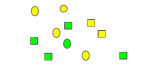Does the formula P(A or B)=P(A)+P(B)-P(A and B) require independance?

marius12

New member
In order to give a representation of the formula:
P(A or B)=P(A)+P(B)-P(A and B)
I just created the following figure:So, here P(green or square)=6/11
But when calculated with the formula P(green)+P(square)-P(green AND square)=(5+6-2)/11=9/11

One problem is that the events green and square are not independent (there are more green square than green circles)

Am I right to say that the formula only work when the events are independent, and that my problem here is precisely that or is it that I do something wrong in my calculations?

ksdhart2

Senior Member
Right off the bat, I see a number of glaring errors. Two seem to come from not paying close enough attention to the drawing, and the other from a fundamental misunderstanding of terms. First, there are four green squares out of eleven total shapes, so $$\displaystyle P(\text{green AND square}) = \dfrac{4}{11} \ne \dfrac{2}{11}$$. Second, there are five green shapes and two yellow squares, making seven shapes that are either green or square (or both), thus $$\displaystyle P(\text{green OR square}) = \dfrac{7}{11} \ne \dfrac{6}{11}$$. Correcting these two errors, we see that:

$$\displaystyle P(\text{green}) + P(\text{square}) - P(\text{green AND square}) = \dfrac{5 + 6 - 4}{11} = \dfrac{7}{11} = P(\text{green OR square})$$

so the supposition holds.

Finally, you appear to have some confusion about what's going on. In this particular instance, whether the two events are independent doesn't matter at all, because you're only picking one shape. Rather, what you need to ask yourself is: Are the events mutually exclusive? That is to say, can both happen at the same time? Obviously the answer to that question is yes - there are two green squares in the drawing.

Strictly speaking, the formula P(A OR B) = P(A) + P(B) - P(A AND B) always holds, but when the two events are mutually exclusive (i.e. both cannot happen at the same time), this necessitates that P(A AND B) = 0, so the formula simplifies down to P(A OR B) = P(A) + P(B).

Jomo

Elite Member
In order to give a representation of the formula:
P(A or B)=P(A)+P(B)-P(A and B)
I just created the following figure:
View attachment 10953
So, here P(green or square)=6/11
But when calculated with the formula P(green)+P(square)-P(green AND square)=(5+6-2)/11=9/11

One problem is that the events green and square are not independent (there are more green square than green circles)

Am I right to say that the formula only work when the events are independent, and that my problem here is precisely that or is it that I do something wrong in my calculations?
Count more carefully! I want to repeat what kshart2 has said as it is very important. The formula P(A OR B) = P(A) + P(B) - P(A AND B) always holds.

Even when you can simplify it to P(A OR B) = P(A) + P(B) because P(A and B) = 0, it is still true that P(A OR B) = P(A) + P(B) - P(A and B)

marius12

New member
Thank you

Thank you very much.
If my problem is only that I have problem to count, then maybe I am not completely lost for mathematics!!!